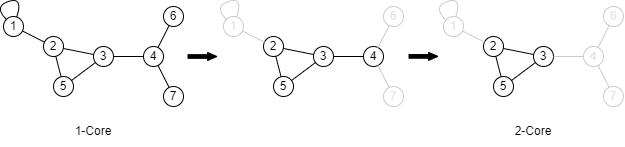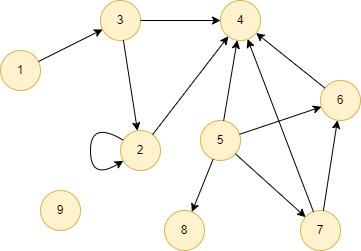# Change Nickname

Current Nickname:

Certifications

Certificate Issued at Valid until Serial No. File
Serial No. Valid until

Not having one? Apply now! >>>

Invoice

ProductName CreateTime ID Price File
ProductName CreateTime ID Price File

# k-Core

## Overview

A k-Core in a graph is a subgraph in which all the nodes in that subgraph have degree no less than k. k-Core algorithm is commonly used to identify and extract the closely connected groups in the graph for further analysis, and are widely used in research fields such as financial risk control, social network and biology due to its low time complexity (liner) and good intuitive interpretability.

## Basic Concept

### k-Core Subgraph

A k-Core of a graph refers to the subgraph generated by iteratively removing nodes whose degree (self-loop edges are ignored) is less than k. The calculation is an iteratively pruning process, nodes with degree no less than k before a certain round of pruning may become less than k after the round.

And once again, in the k-Core algorithm, self-loop edges of node have no contribution to the degree of the node.Start from the graph on the left, the minimum degree of all nodes is 1, so the graph is 1-Core of itself; in order to obtain the 2-Core, all nodes with degree equals to 1, which are `1`, `6` and `7`, are removed, so that the graph in the middle. And after removing nodes `6` and `7`, the degree of node `4` decreases to 1, thus it has to be removed too. The rest nodes in the subgraph on the right all have degree of 2, so the 2-Core of the original graph contains nodes `2`, `3` and `5`.

### Coreness

If a node belongs to the k-Core of a graph, but it is not included in the (k+1)-Core, then this node is considered to have the coreness of k.

In the previous example, 1-Core contains nodes [1,2,3,4,5,6,7], 2-Core contains nodes [2,3,5], 3-Core does not exist. So nodes [1,4,6,7] has coreness of 1, nodes [2,3,5] has coreness of 2.

## Special Case

### Lonely Node, Disconnected Graph

Lonely node is not connected with other nodes, the degree of lonely node is 0 in the calculation of k-Core.

The connectivity of the graph does not affect the calculation of k-Core, the originally connected graph might become disconnected during the calculation of k-Core.

### Self-loop Edge

Self-loop edge does not participate in the calculation of k-Core.

### Directed Edge

For directed edges, k-Core algorithm ignores the direction of edges but calculates them as undirected edges.

## Results and Statistics

Take the graph below as an example, run the k-Core algorithm:Algorithm results: Given k=3, return the node list of its 3-Core subgraph






Algorithm statistics: N/A

## Command and Configuration

• Command: `algo(k_core)`
• Configurations for the parameter `params()`:
Name Type Default Value Specification Description
k int / >0; mandatory Coreness K

## Algorithm Execution

#### 1. File Writeback

Configuration Data in Each Row
filename `_id`

Example: Calculate 3-Core subgraph, write the algorithm results back to file named 3-core

``````algo(k_core).params({
k:3
}).write({
file:{
filename: "3-core"
}
})
``````

#### 2. Property Writeback

Not supported by this algorithm.

#### 3. Statistics Writeback

Not supported by this algorithm.

### Direct Return

Alias Ordinal Type Description Column Name
0 []perNode Nodes in k-Core `_uuid`

Example: Calculate 2-Core subgraph, define algorithm results as alias named k2, return the results

``````algo(k_core).params({
k:2
}) as k2
return k2
``````

### Streaming Return

Alias Ordinal Type Description Column Name
0 []perNode Nodes in k-Core `_uuid`

Example: Calculate 2-Core subgraph, define algorithm results as alias named k2, return the number of nodes in the 2-Core subgraph

``````algo(k_core).params({
k:2
}).stream() as k2
return count(k2)
``````

### Real-time Statistics

This algorithm has no statistics.